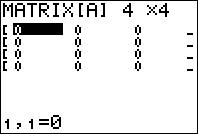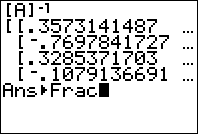﻿ Finding the Inverse of a Matrix with a Graphing Calculator

# Finding the Inverse of a Matrix with the TI83 / TI84

By taking any advanced math course or even scanning through this website, you quickly learn how powerful a graphing calculator can be. A more “theoretical” course like linear algebra is no exception. In fact, once you know how to do something like finding an inverse matrix by hand, the calculator can free you up from that calculation and let you focus on the big picture.

Remember, not every matrix has an inverse. The matrix picked below is invertible, meaning it does in fact have an inverse. We will talk about what happens when it isn’t invertible a little later on. Here is the matrix we will use for our example:

$$\left[ \begin{array}{cccc} 8 & 2 & 1 & 6 \\ 8 & 4 & 1 & 1 \\ 0 & 2 & 6 & 4 \\ 15 & 8 & 9 & 20 \end{array} \right]$$

Note: for a video of these steps, scroll down.

### Step 1: Get to the Matrix Editing Menu

This is a much more involved step than it sounds like! If you have a TI 83, there is simply a button that says “MATRIX”. This is the button you will click to get into the edit menu. If you have a TI84, you will have to press [2ND] and [$$x^{-1}$$]. This will take you into the menu you see below. Move your cursor to “EDIT” at the top.Now you will select matrix A (technically you can select any of them, but for now, A is easier to deal with). To do this, just hit [ENTER].### Step 2: Enter the Matrix

First, you must tell the calculator how large your matrix is. Just remember to keep it in order of “rows” and “columns”. For example, our example matrix has 4 rows and 4 columns, so I type 4 [ENTER] 4 [ENTER].Now you can enter the numbers from left to right. After each number, press [ENTER] to get to the next spot.Now, before we get to the next step. On some calculators, you will get into a strange loop if you don’t quit out of this menu now. So, press [2ND] and [MODE] to quit. When you do this, it will go back to the main screen.

### Step 3: Select the Matrix Under the NAMES Menu

After you have quit by clicking [2ND] and [MODE], go back into the matrix menu by clicking [2ND] and [$$x^{-1}$$] (or just the matrix button if you have a TI83). This time, select A from the NAMES menu by clicking [ENTER].### Step 4: Press the Inverse Key [$$x^{-1}$$] and Press Enter

The easiest step yet! All you need to do now, is tell the calculator what to do with matrix A. Since we want to find an inverse, that is the button we will use.At this stage, you can press the right arrow key to see the entire matrix. As you can see, our inverse here is really messy. The next step can help us along if we need it.

### Step 5: (OPTIONAL) Convert Everything to Fractions

While the inverse is on the screen, if you press [MATH] , 1: Frac, and then ENTER, you will convert everything in the matrix to fractions. Then, as before, you can click the right arrow key to see the whole thing.That’s it! It sounds like a lot but it is actually simple to get used to. It’s useful too – being able to enter matrices into the calculator lets you add them, multiple them, etc! Nice! If you want to see it all in action, take a look at the video to the right where I go through the steps with a different example. Even with the optional step, it takes me less than 3 minutes to go through.

Oh yeah – so what happens if your matrix is singular (or NOT invertible)? In other words, what happens if your matrix doesn’t have an inverse?As you can see above, your calculator will TELL YOU. How nice is that?

## Video walkthrough

The following video will walk you through the steps above.